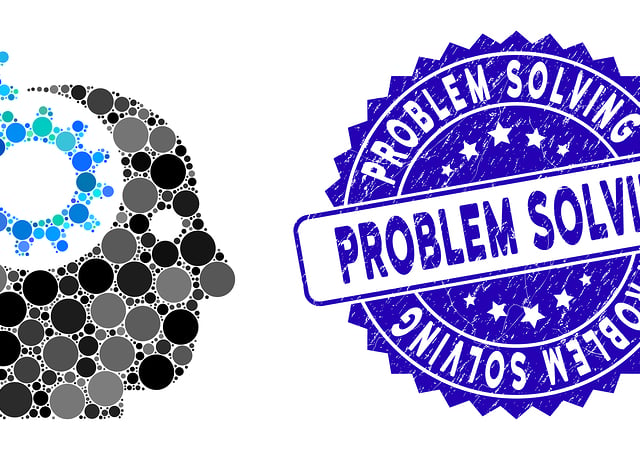# Formula Chart of the SAT® Problem Solving and Data Analysis Questions

If you thought math wasn’t about everyday life, then the SAT® Problem Solving and Data Analysis questions are going to change your mind! These questions are all about solving problems you’ll really face in the future, such as finding rates, understanding graphs, calculating interest rates, and so on.

There will be 15 questions of this type on the SAT® Test. What should you do to succeed on the SAT® Problem Solving and Data Analysis questions? Well, you could start by reading our free study guide, solving our free practice test, and learning all the essential formulas you’ll need, provided in the formula chart, below.

To see formulas used in the other three areas of math on the SAT®, check out our charts for those:

Here are the Problem-Solving and Data Analysis formulas you’ll need:

Category Formula Symbols Comment
Problem
Solving and
Data Analysis
$$Du=Su \cdot \dfrac{Du}{Su}=Su \cdot CF$$ Du = Desired Unit
Su = Starting Unit
CF = Conversion Factor
Multiple steps may
be needed.
Problem
Solving and
Data Analysis
$$a \cdot b\% =a \cdot \frac{b}{100}$$ a = any real number
b% = any percent
Remember to simplify
if necessary
Problem
Solving and
Data Analysis
$$\% = \frac{\vert b-a \vert }{b} \cdot 100= \frac{c}{b} \cdot 100$$ % = % increase or decrease
a = new value
b = original value
c = amount of change

Problem
Solving and
Data Analysis
$$rg=lv -sv$$ rg = range of data
lv = largest value in the data set
sv = smallest value in the data set

Problem
Solving and
Data Analysis
$$\overline{x}= \dfrac{\Sigma x_i}{n}$$ $$\overline{x}$$ = mean
$$x_i$$ = value of each measurement
n = number of measurements

Problem
Solving and
Data Analysis
$$Md=(\dfrac{n+1}{2})^{th} term$$ Md = median
n = number of measurements (odd)

Problem
Solving and
Data Analysis
$$Md=\dfrac{(\frac{n}{2})^{th} term + (\frac{n+1}{2})^{th} term }{2}$$ Md = median
n = number of measurements (even)

Problem
Solving and
Data Analysis
$$s=\sqrt{\Sigma(x_i-\overline{x})^2/(n-1)}$$ s = standard deviation
$$\overline{x}$$ = mean
$$x_i$$ = value of each measurement
n = number of measurements

Problem
Solving and
Data Analysis
$$V=s^2$$ v = Variance
s = standard deviation

Problem
Solving and
Data Analysis
$$CV=RSD=100 \cdot \dfrac{s}{\overline{x}}$$ CV = Coefficient of variation
RSD = Relative standard deviation
s = standard deviation

Problem
Solving and
Data Analysis
$$SI=P \cdot IR \cdot t$$ SI = Simple interest
P = Principal (Amount borrowed)
IR = Interest rate
t = time (use same time units as in IR)

Problem
Solving and
Data Analysis
$$A_{SI}=P + SI = P \cdot (1+IR \cdot t)$$ $$A_SI$$ = Future value to be paid (for SI)
P = Principal (Amount borrowed)
SI = Simple interest
IR = Interest rate
t = time (use same time units as in IR)
For simple interest
Problem
Solving and
Data Analysis
$$A_{CI} = P \cdot (1+ \dfrac{IR}{n})^{n \cdot t}$$ $$A_CI$$ = Future value to be paid (for CI)
= P = Principal (Amount borrowed)
IR = Interest rate
n = num. of times int. is compounded per unit time
t = time (use same time units as in IR)
For compound interest